# Analysis and Simulation of PCB ACR Losses for Vcore DrMOS Optimization

### by Eric Braun, Staff Scientist; Jinghai Zhou, Director of Technical Marketing & Applications Engineering; Danny Chang, Sr. Manager of Technical Marketing & Applications Engineering, Monolithic Power Systems (MPS); and Huaifeng Wang, Design Development Engineering Manager, Huawei

Printed circuit board (PCB) main-loop ripple current resistive losses are often neglected and overlooked as a switching power supply loss component. These losses can be significant for high-current Vcore and other applications that operate with high ripple current. Ansys Q3D is used to extract the main loop ripple current frequency-dependent resistance for a typical Vcore power stage PCB layout. Including this loss component significantly improves the correlation between the modeled and measured total loss as a function of the switching frequency. An analytic expression is developed to optimize the switching frequency for peak efficiency at the balance point between the negative frequency coefficient PCB and passive component losses and the positive frequency coefficient MOSFET conventional switching losses.

### Introduction

Past studies that have modeled buck converter power losses focused primarily on semiconductor device losses1,2,3,4,5, and to a lesser extent, passive component losses1,2, while PCB losses generally and PCB ACR losses specifically have been largely ignored. To estimate these losses, Ansys Q3D is used to extract the PCB main loop ACR for a typical Vcore DrMOS application, from which the associated ripple current ACR losses are calculated. These losses increase non-linearly with a decreasing frequency due to the increasing peak-to-peak ripple current, resulting in lower peak efficiency at a higher switching frequency than in the conventional loss analysis prediction.

### PCB Main Loop ACR Model, Simulation, and Measurement

The major power-loss components of synchronous buck converters can be summarized as active component losses (MOSFET DC and switching losses, MOSFET driver losses) passive component losses (inductor DCR, ACR and core losses, capacitor ESR losses), and printed circuit board losses (PCB DCR and ACR losses).

The frequency-dependent PCB losses are due to the sawtooth-shaped ripple current that circulates in the main loop of the converter given by Equation (1):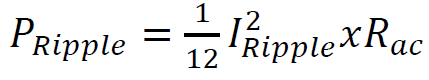(1)

Where Rac is the frequency-dependent PCB effective resistance of the ripple current waveform. Vcore DrMOS synchronous buck converters with a relatively large ripple current under peak efficiency operating conditions provide a case to study. To estimate the PCB ACR loss component, a single-phase portion of a multi-phase Vcore PCB power stage layout was imported into Anysis Q3D (see Figure 1a). The Q3D simulated AC current distribution in the PCB main loop ripple current circulation path is shown in Figure 1b.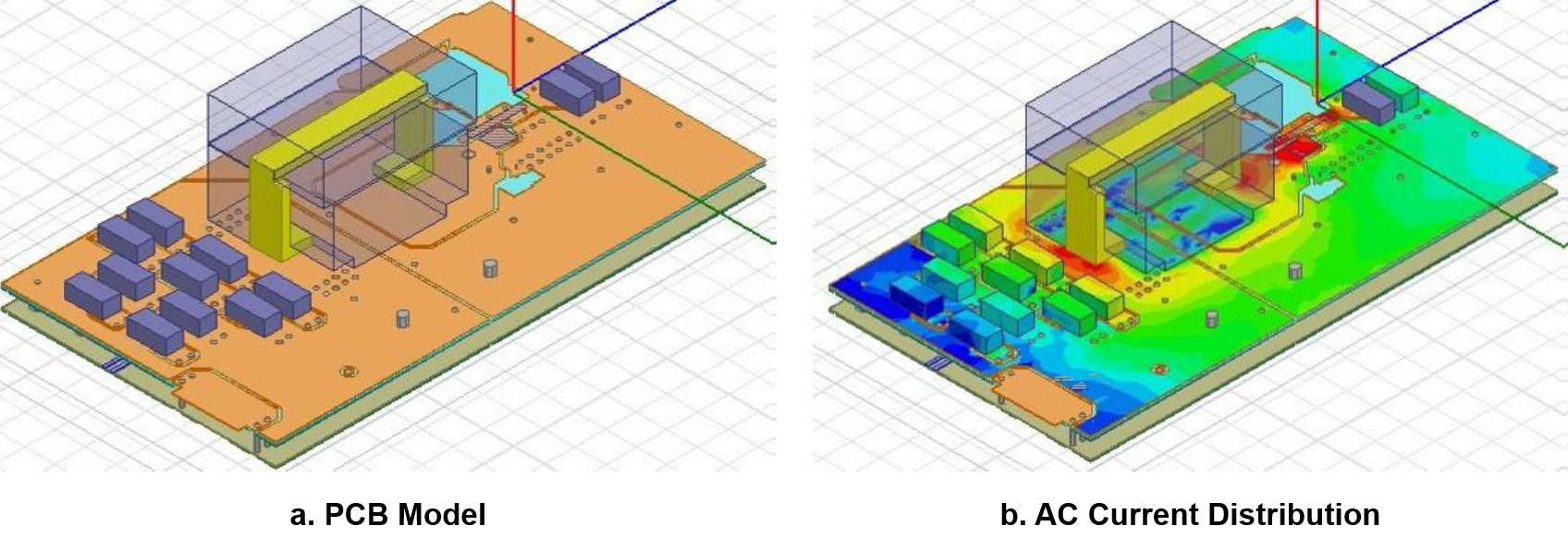Figure 1: Vcore PCB Main Loop Q3D Structure Model and AC Current Simulation

The Q3D frequency-dependent resistance extraction closely matches the board level LCR measurement shown in Figure 2. Additionally, the PCB frequency-dependent resistance reasonably follows the ideal skin effect model shown in Equation (2):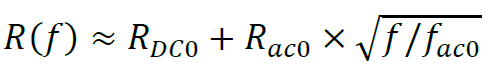(2)

The effective ripple current resistance (Rac) for the power stage sawtooth waveform power loss calculation is estimated by weighted Fourier analysis applied to the 15% duty cycle triangle wave, resulting in an equivalent power loss calculation ACR approximately equal to 1.1 times the simulated fundamental switching frequency component ACR.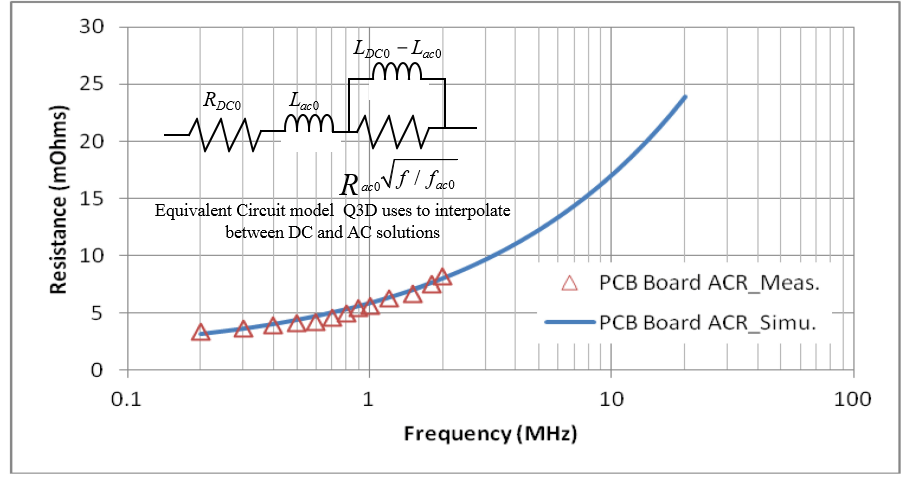Figure 2: Simulated and Measured Vcore PCB Main Loop Frequency Dependent Resistance (ACR)

### Total Converter Loss Model vs Measurement

The total switching loss measurement was made on a single-phase Vcore DrMOS evaluation board utilizing the recommended 150nH inductor at the typical operating conditions of Vin = 12V and Vout = 1.8V with the resulting peak-to-peak ripple current (Ipp) ~14.5A at 700kHz. The power losses of the converter at 15A load current were analyzed over the frequency range of 400kHz to 2.5MHz with loss components extracted at 700kHz (see Figure 3 and Figure 4). MOSFET losses were estimated by a combination of device measurement and simulation. Inductor winding ACR loss was based on Q3D simulation, while inductor core losses and DCR losses were estimated based on the supplier's datasheet. Output POSCAP ESR loss was extracted based on the measured power loss difference replacing the POSCAPs with ceramic ones. PCB ACR loss was derived from the Q3D frequency dependent analysis described above. The PCB ACR loss accounted for ~25% of the ripple current resistive losses and ~5% of the total loss at the peak efficiency operating frequency of 700kHz.Figure 3: Vcore DrMOS Power Loss Constituents at 700kHz

The overall power loss can be expressed in Equation (3):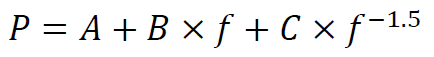(3)

Where A is the DC power loss, B × f is the proportional-to-frequency MOSFET switching power loss, and C × f −1.5 is the ripple current resistive power loss. When solving for the minimum overall power loss, the peak efficiency frequency is found when the proportional-to-frequency MOSFET power loss equals 1.5 times the ripple current power loss. This crossover occurs at approximately 700kHz for the Vcore DrMOS operating conditions under study (see Figure 4).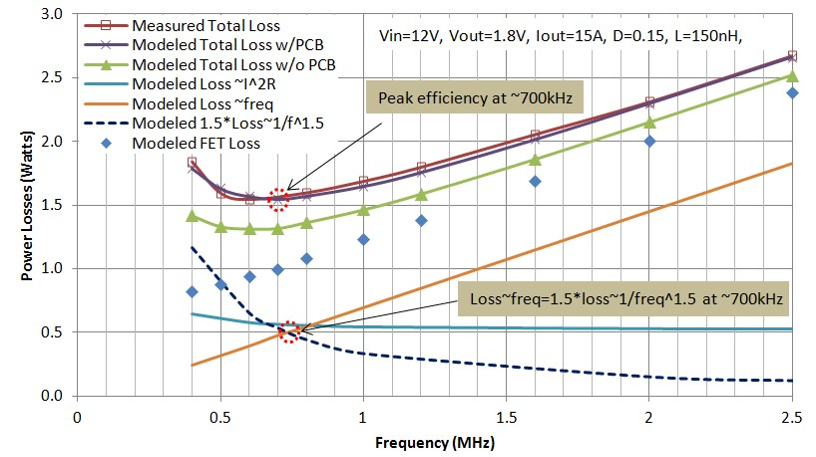Figure 4: Vcore DrMOS Power Losses vs Frequency

### Conclusion

Main-loop ripple current PCB resistive losses can be a significant loss component, limiting peak efficiency substantially below conventional analysis estimation methods. These losses can be reasonably estimated using Q3D resistance extraction for improved efficiency versus frequency estimation. The peak efficiency operating frequency is found to be higher than without including the PCB ACR loss factor. Owing to the monolithic integration of drivers and MOSFETs, MPS DrMOS devices are inherently capable of higher frequency operation to mitigate this loss factor.

1 Gregory Sizikov, Edy G. Fridman, and Michael Zelikson, “Efficiency Optimization of Integrated DC-DC Buck Converters,” IEEE ICECS, 2010, pp. 1208-1211.
2 Volkan Kursun, Siva G. Narendra, Vivek K. De, and Eby G. Friedman, “Efficiency Analysis of High Frequency Buck Converter for On-Chip Integration with a Dual-VDD Microprocessor,” ESSCIRC, 2002, 743-746.
3 Yuancheng Ren, Ming Xu, Jinghai Zhou, Fred C. Lee, “Analytical Loss Model of Power MOSFET,” IEEE, Power Electronics, Vol.21 NO.2, 2006 , pp. 310-319.
4 Wison Eberle, Zhiliang Zhang, Yan-Fei Liu, and Paresh C. Sen, “A Practical Switching Loss Model for Buck Voltage Regulators,” IEEE, Power Electronics, Vol.24 NO.3, 2009 , pp. 700-712.
5 Yali Xiong, Shan Sun, Hongwei Jia, Patrick Shea, and Z. John Shen, “New Physical Insights on Power MOSFET Switching Losses,” IEEE, Power Electronics, Vol.24 NO.2, 2009 , pp. 525-531.

___________________________________

## Technical Forum

Get technical support